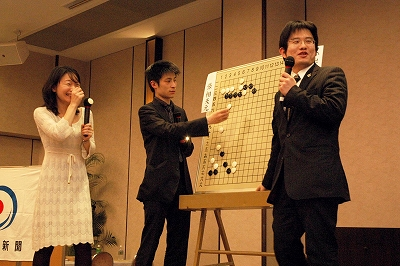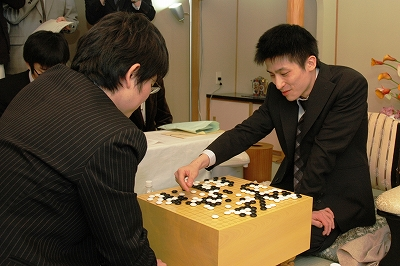## Posts Tagged ‘tengen’

### Yamashita Keigo Captured Tengen Title from Cho U

Tuesday, December 22nd, 2009

Yamashita Keigo won the fifth game in the best-of-five Tengen title match and captured the title from Cho U with a 3:2 score.This title match must have been very tensed especially since these two will meet again very soon in an even more important battle: the first game of the Kisei title starts on January 14th with Cho as challenger, and Yamashita as the Kisei title holder.

Cho U had mixed results this year: he lost the Meijin and Tengen titles, but defended Gosei and Oza. Will he be able to capture Kisei for the first time, especially from Yamashita who has been Kisei for four consecutive years?

The 5th and decisive Tengen title game record is here. Yamashita Keigo (black) won by resignation.

(;GMFFCA[UTF-8]AP[CGoban:3]ST RU[Japanese]SZKM[6.50] GN[35th Tengen Title, game 5]PW[Cho U]PB[Yamashita Keigo]WR[9p]BR[9p]DT[2009-12-21]EV[35th Tengen Title, game 5]RO[Title match]RE[B+Resign]MULTIGOGM ;B[pd] ;W[dd] ;B[qp] ;W[op] ;B[lp] ;W[qo] ;B[ro] ;W[po] ;B[rn] ;W[qq] ;B[rq] ;W[pp] ;B[rp] ;W[pl] ;B[qr] ;W[dq] ;B[do] ;W[cm] ;B[cq] ;W[gq] ;B[dp] ;W[dr] (;B[iq] ;W[bo] ;B[bp] ;W[co] ;B[cp] ;W[go] ;B[dm] ;W[cl] (;B[gm] ;W[io] ;B[kq] (;W[hm] (;B[hl] (;W[fm] (;B[fp] ;W[hq] ;B[fl] (;W[gn] ;B[gl] (;W[ln] (;B[im] ;W[ir] (;B[no] (;W[on] (;B[mm] ;W[mn] ;B[nn] ;W[nm] ;B[nl] ;W[om] ;B[np] ;W[nq] ;B[mq] ;W[ml] ;B[lm] ;W[km] ;B[ll] ;W[mk] ;B[kl] ;W[oj] ;B[cj] ;W[dj] ;B[ci] ;W[ck] ;B[em] ;W[di] ;B[cg] ;W[ch] ;B[bh] ;W[dh] ;B[dg] ;W[bi] ;B[bj] ;W[ai] ;B[jr] ;W[ip] ;B[jq] ;W[cr] ;B[er] ;W[eq] ;B[fq] ;W[fr] ;B[gr] ;W[es] ;B[hr] ;W[ep] ;B[fo] ;W[eo] ;B[fn] ;W[dn] ;B[bf] ;W[bk] ;B[cc] ;W[dc] ;B[cd] ;W[hc] ;B[lj] ;W[mj] ;B[jc] ;W[nc] ;B[oc] ;W[nd] ;B[pf] ;W[ie] ;B[ke] ;W[kj] ;B[li] ;W[lk] ;B[kk] ;W[ng] ;B[kh] ;W[kd] ;B[ld] ;W[jd] ;B[lc] ;W[le] ;B[me] ;W[lf] ;B[mf] ;W[mg] ;B[lg] ;W[kf] ;B[jf] ;W[je] ;B[kg] ;W[ke] ;B[oe] ;W[md] ;B[ne] ;W[kc] ;B[lb] ;W[mb] ;B[mi] ;W[ni] ;B[pi] ;W[jj] ;B[ik] ;W[pj] ;B[oh] ;W[ki] ;B[ig] ;W[lh] ;B[mh] ;W[nh] ;B[lh] ;W[ce] ;B[be] ;W[qh] ;B[qi] ;W[og] ;B[pg] ;W[ph] ;B[rh] ;W[qg] ;B[rg] ;W[qf] ;B[qe] ;W[ij] ;B[gg] ;W[gi] ;B[gh] ;W[jk] ;B[jl] ;W[hk] ;B[fi] ;W[il] ;B[jm] ;W[hn] ;B[ik] ;W[ih] ;B[hg] ;W[il] ;B[kn] ;W[fj] ;B[gj] ;W[gk] ;B[ej] ;W[fk] ;B[ok] ;W[ol] ;B[nk] ;W[nj] ;B[qm]) (;B[nn] ;W[np]) (;B[np] ;W[mm] ;B[oq])) (;W[nn] ;B[on] ;W[nm] ;B[oo] ;W[mo] ;B[np] ;W[mp] ;B[mq] ;W[nq] ;B[oq] ;W[nr] ;B[pn] ;W[mr] ;B[lq] ;W[js] ;B[jp])) (;B[cj] ;W[dj] ;B[ci] ;W[ck] (;B[em] ;W[di] ;B[cg] ;W[dh] ;B[bf] ;W[ce] ;B[dg] ;W[eg] ;B[ef] ;W[fg]) (;B[di] ;W[em] ;B[dl] ;W[el] ;B[dk] ;W[dn])) (;B[ip] ;W[in] ;B[jr])) (;B[dl]) (;B[dj]) (;B[cj])) (;W[em] ;B[dl] (;W[dn] ;B[en] ;W[cn] ;B[fn]LB[eo:A]SQ[fp]C[The square marked black stone protects against white's cut at A.]) (;W[el] ;B[dk] ;W[ek] ;B[ej] ;W[fk] ;B[dn] ;W[ln]))) (;W[em] ;B[dl] ;W[el] ;B[dk] ;W[fk] ;B[ck] ;W[gl])) (;B[fl] ;W[gn] ;B[gl] ;W[em] (;B[fp]) (;B[dl] ;W[dn] ;B[en] ;W[cn] ;B[fn] ;W[eo]))) (;W[im] ;B[gn] ;W[ho] ;B[lm]) (;W[gl] ;B[fm] (;W[hk] ;B[il] ;W[im] ;B[dl]) (;W[en] ;B[dn] ;W[em]))) (;B[gl] ;W[ln])) (;W[hq]) (;W[jr]) (;W[ek] ;B[im])) (;B[fm]) (;B[dl])) (;B[go]))

The complete tournament table is here.

### Yamashita Keigo – Cho U 2:1 in Tengen

Wednesday, December 2nd, 2009

Yamashita Keigo won the third game from the Tengen title match, and leads title-holder Cho U 2:1.The game record is here. Yamashita Keigo (black) won by resignation.

(;CA[Windows-1252]SZAP[MultiGo:4.4.4]GN[Tengen Title 2009]EV[Tengen Title 2009] DT[2009-12-02]PB[Yamashita Keigo]PW[Cho U]KM[6.5]HARE[B+R]MULTIGOGM ;B[qd];W[pp];B[dd];W[cp];B[ep];W[gq];B[cq];W[cm];B[dn];W[bo];B[fq];W[gp];B[bq];W[cj] ;B[oc];W[qn];B[pj];W[fc];B[hc];W[df];B[fd];W[cc];B[cd];W[dc];B[ed];W[fb];B[gc];W[bc] ;B[nf];W[gd];B[id];W[ge];B[ef];W[gg];B[eh];W[gi];B[ej];W[di];B[fh];W[gh];B[fj];W[hk] ;B[dm];W[cl];B[dk];W[je];B[ie];W[jg];B[if];W[kh];B[ff];W[nh];B[ph];W[og];B[nj];W[pg] ;B[qg];W[qf];B[qh];W[pe];B[nd];W[re];B[rd];W[me];B[ne];W[mi];B[cn];W[dl];B[bn];W[el] ;B[gf];W[qk];B[mj];W[kk];B[kj];W[pd];B[pc];W[rc];B[qc];W[lj];B[pk];W[lk];B[ql];W[oq] ;B[kq];W[iq];B[mq];W[mo];B[lo];W[ln];B[ko];W[mp];B[lp];W[rl];B[qm];W[rm];B[pn];W[qj] ;B[qo];W[rn];B[po];W[pm];B[pl];W[om];B[nn];W[nm];B[mn];W[mm];B[on];W[ri];B[lm];W[ml] ;B[ro];W[rq];B[rh];W[oi];B[pi];W[sn];B[si];W[sj];B[qi];W[sh];B[sg];W[np];B[jm];W[sf] ;B[si];W[rj];B[rp];W[sh];B[qq])

Next game will be played on December 10th. You can find the complete tournament table here.

### Cho U Evens the Score in Tengen Title Match

Thursday, November 19th, 2009

Cho U, Tengen title holder, defeated the challenger Yamashita Keigo in the second game.The game record is here. Cho U, playing black, won by resignation.

(; KM[6.5] EV[35th Tengen title match 1] FF SZ GM PW[Yamashita Keigo] WR[Kisei] DT[2009-11-19] BR[Tengen] RE[B+R] PB[Cho U] ;B[pd];W[dp];B[qp];W[dc];B[fq];W[cn];B[lq];W[de];B[pj];W[nc];B[lc] ;W[qc];B[qd];W[pc];B[od];W[nd];B[oc];W[ob];B[pb];W[qb];B[nb];W[pa] ;B[ne];W[mb];B[me];W[ip];B[fo];W[in];B[dr];W[cq];B[fm];W[il];B[op] ;W[gp];B[ep];W[en];B[fn];W[gq];B[do];W[co];B[dq];W[cp];B[jo];W[jn] ;B[ko];W[kq];B[kr];W[jq];B[lp];W[fr];B[em];W[dn];B[eo];W[fk];B[dl] ;W[bl];B[ck];W[bk];B[gk];W[gl];B[io];W[hn];B[ho];W[go];B[gn];W[jr] ;B[er];W[gr];B[di];W[fi];B[bj];W[cg];B[lr];W[ir];B[dg];W[ch];B[dh] ;W[df];B[ci];W[fg];B[ek];W[fl];B[ej];W[fj];B[br];W[cr];B[cs];W[bq] ;B[bf];W[cf];B[bh];W[rp];B[ro];W[qo];B[po];W[qn];B[rq];W[oh];B[qh] ;W[of];B[qf];W[jd];B[mc];W[na];B[nj];W[mh];B[md];W[ql];B[qk];W[rk] ;B[rj];W[ok];B[oj];W[om];B[nl];W[pk];B[qj];W[pn];B[lj];W[oo];B[pp] ;W[mk];B[nk];W[rn];B[sp];W[sl];B[ol];W[pl];B[no];W[on];B[sn];W[rm] ;B[sj];W[sk];B[je];W[ie];B[jf];W[li];B[id];W[jc];B[jh];W[ig];B[if] ;W[he];B[hf];W[ge];B[kj];W[bg];B[ag];W[mj];B[ll];W[ki];B[ji];W[jj] ;B[kl];W[jg];B[kg];W[kf];B[hg];W[ih];B[kh];W[lf];B[hi];W[ke];B[kd] )

Next game will be played on December 3rd.

### Tengen 2009: Yamashita Wins Game One

Thursday, November 12th, 2009

Yamashita Keigo, challenger for this year Tengen title, won game one against title-holder Cho U.Here is the game record. Cho U (white) resigned after black’s 281 move.

(; EV[35th Tengen title match 1] KM[6.5] FF SZ GM PW[Cho U] WR[Tengen] PB[Yamashita Keigo] BR[Kisei] RE[B+R] DT[2009-11-12] ;B[pd];W[dd];B[qp];W[op];B[oo];W[qq];B[pp];W[pq];B[no];W[np];B[mp] ;W[nr];B[mr];W[mq];B[lq];W[nq];B[lp];W[po];B[pn];W[qo];B[rp];W[rn] ;B[rq];W[qm];B[or];W[lr];B[oq];W[ms];B[os];W[kr];B[ns];W[mr];B[qr] ;W[mo];B[nm];W[mn];B[ko];W[mm];B[nl];W[iq];B[qk];W[qj];B[pk];W[rk] ;B[pj];W[rj];B[qh];W[ml];B[qi];W[pl];B[ol];W[ql];B[ok];W[ri];B[rh] ;W[si];B[km];W[kl];B[jl];W[jm];B[jn];W[im];B[kk];W[ll];B[in];W[jk] ;B[il];W[hm];B[hn];W[ik];B[hl];W[gm];B[hk];W[hj];B[fl];W[fm];B[kj] ;W[ij];B[fk];W[mj];B[hp];W[gq];B[hq];W[hr];B[em];W[fo];B[gr];W[fr] ;B[en];W[gp];B[go];W[ip];B[ho];W[fj];B[fn];W[lh];B[ej];W[fi];B[ei] ;W[eh];B[fh];W[dh];B[gh];W[hi];B[kh];W[ek];B[el];W[dk];B[kg];W[nn] ;B[on];W[lg];B[lf];W[li];B[ki];W[mf];B[ni];W[om];B[pm];W[qn];B[om] ;W[nk];B[oi];W[le];B[kf];W[ng];B[me];W[ne];B[md];W[pf];B[qf];W[pe] ;B[qe];W[pc];B[qc];W[ph];B[oh];W[od];B[qd];W[kd];B[mc];W[ob];B[pb] ;W[oc];B[jd];W[jc];B[ke];W[id];B[ld];W[qb];B[rb];W[pa];B[kc];W[pg] ;B[pi];W[ra];B[nj];W[fc];B[og];W[of];B[lj];W[cd];B[cj];W[dj];B[ck] ;W[cp];B[eq];W[bm];B[cl];W[gs];B[cq];W[bq];B[dq];W[br];B[do];W[co] ;B[cg];W[ic];B[hg];W[be];B[kq];W[jq];B[bn];W[cn];B[cm];W[bl];B[bo] ;W[bp];B[bk];W[ao];B[fd];W[gd];B[ge];W[ed];B[fe];W[dg];B[bg];W[he] ;B[hf];W[if];B[ig];W[ee];B[sk];W[sl];B[mi];W[rc];B[rd];W[sb];B[gc] ;W[hd];B[fb];W[ec];B[kb];W[gb];B[ia];W[ib];B[ga];W[hb];B[ja];W[ha] ;B[mg];W[nf];B[nh];W[fg];B[gf];W[ch];B[bh];W[fp];B[eo];W[cf];B[nb] ;W[ff];B[cr];W[so];B[nd];W[sh];B[er];W[bf];B[bs];W[bi];B[ci];W[di] ;B[ai];W[sf];B[je];W[ie];B[sd];W[jo];B[kp];W[ag];B[bj];W[nc];B[na] ;W[sp];B[sq];W[oa];B[mb];W[jb];B[ka];W[ih];B[rf];W[sc];B[hh];W[af] ;B[ak];W[an];B[ah];W[fq];B[es];W[fs];B[sg];W[rg];B[qg];W[oe];B[sg] ;W[cs];B[ds];W[rg];B[jr];W[ir];B[sg])

Cho U has captured the Tengen title from Kono Rin last year, after Yamashita Keigo has unsuccessfully challenged Kono Rin for two years in a row.

Tournament table here.

### Cho U Captures Tengen Title

Wednesday, December 3rd, 2008
Cho U defeated Kono Rin 3-0 in his Tengen title challenge. Cho won the third game by resignation. He owns now Meijin, Tengen and Gosei among the “big seven” titles, and leads 2-1 in his Oza challenge. Here is the game record of the 3rd game in Tengen.

### Cho U 2-0 in Tengen Title Challenge

Saturday, November 22nd, 2008

Cho U won the second game as well in his challenge for the Tengen title against Kono Rin. This victory brings him just one victory away from capturing Tengen. Third game will be played on December 4th.

Here is the game record. I was very impressed by move 141 made by Cho U: it’s a killing move that defies the “Don’t attach against stones you are attacking”. On second though, maybe it is not defying it at all, since this is not an attacking move, but a killing move…

Also, here is the game record from the first game.

### Kono Rin defends Tengen title

Sunday, December 16th, 2007
I got asked in this blog comment: “How about covering the Tengen?” I started writing a belated reply, when I realized that I’d better turn it into a blog entry. Here it is: The reason I didn’t cover Tengen (and other recent tournaments) on the website is because I realized that I don’t have the resources (and by resources I mean “time”) to keep track of all current titles and tournaments. I did follow Tengen myself though – it was especially interesting for me because Kono Rin (he is the Tengen titleholder, he just defended it against Yamashita Keigo Kisei) was insei when I was (he kicked my butt by 2.5 points in the only game we played – since he was mostly 1-2 classes above me.) He was still insei when I quit (he became pro 1 or 2 years later) and I would have never guessed that he’ll become a title holder. His style, just like his personality, was very quiet, deep-thinking type, never spectacular or attracting attention. He was very serious all the time, I am sure he must have studied very-very hard.